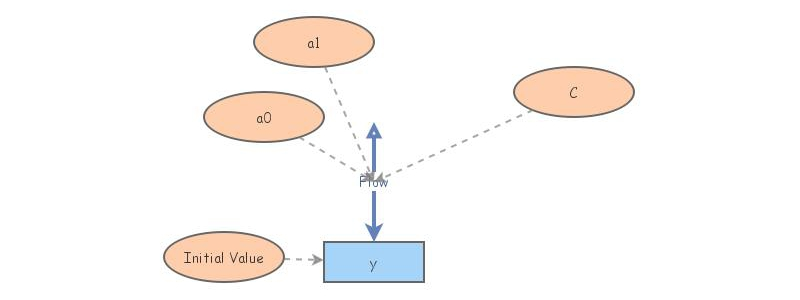Modified Lotka-Volterra EquationThis models the differential equation dy/dt = a0+a1 y*(y/C-1) for different non-negative values of a0, a1, and C.

In order for there to be a stable fixed point, C*a1 needs to be bigger than or equal to 4*a0. Running the simulation for values of C*a1 close to 4*a0 will result in inaccurate values due to numerical errors in the approximation methods used. To get accurate results, it is best to run the simulation with C*a1 > 4*a0.

Setting a0 = 2, a1 = 3, and C = 3 produces a stable fixed point at y = 1, and an unstable fixed point at y = 2.